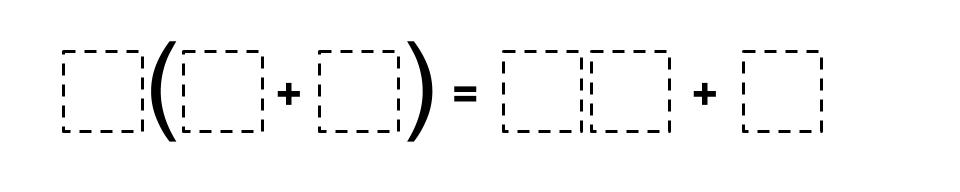Home > Grade 6 > Expressions & Equations > Distributive Property

# Distributive Property

Directions: Fill in the boxes below using the whole numbers 0 through 9 no more than one time each so that you can make a true equation.### Hint

What numbers would you need in the parentheses to give you a two digit number and a one digit number when you distribute?

There are many possible answers. One possible answer: 2(9 + 3) = 18 + 6

## Subtracting Multi-Decimals

Directions: Use the digits 1 to 9, at most one time each, to fill in …

1.2(5+4) = 10 + 8

Going to try this with my students after having studied and reviewed the distributive property with algebra tiles. I’ll let you know how it goes!

2.I’m not so sure there are all that many possible answers. I convinced myself (though I’m not 100% sure yet) that there are only 4: the two already listed, and also 2(7+3) = 14 + 6 and 2(5+3)=10 + 6.

•In addition to the 4 that are now listed I also have 4(9+2) = 36 +8 as a solution.
These are the answers I come up with if I want both sides of the equation to simply show distributing and factoring of numbers.
There are, however, more possible answers if we open up the problem to any equivalent statements.
For example: 5(3+4) = 28 + 7
Both sides of the equation equal 35 (if I did my math correctly). I haven’t made a list of all of those possibilities but I imagine there are quite a few more. I have found that it depends on how I frame the problem the types of answers I get. If students know it is to work on distributive property the solutions they come up with are the 5 we have listed. If I don’t mention the distributive property then I get both types of solutions.

3.Since you can use 0 as a digit, that opens up two more possible answers:
2(4+3) = 08 + 6, and 2(3+4) = 06 + 8

•